# Determinant of transpose

An important fact in linear algebra is that, given a matrix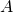,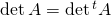, where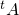is the transpose of. Here I will prove this statement via explciit computation, and I will try to do this as cleanly as possible. We may define the determinant ofby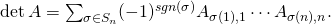Here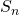is the set of permutations of the set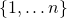, and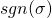is the sign of the permutation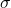. This formula is derived from the definition of the determinant via exterior algebra. One can check by hand that this gives the familiar expressions for the determinant when.

Now, since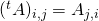, we have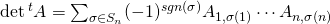The crucial observation here is that we may rearrange the product inside the summation so that the second indices are increasing. Let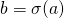. Then the product inside the summation isCombining this with the fact that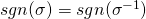, our expression simplifies to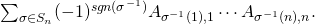Noticing that the sum is the same sum if we replace all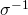s withs, we see that this equals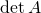. So.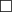I wonder if there is a more conceptual proof of this? (By “conceptual”, I mean a proof based on exterior algebra, bilinear pairings, etc…)